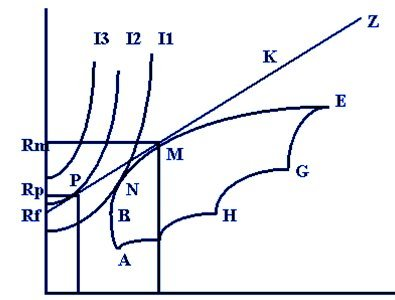# Selecting Optimal Portfolio for an Investor

In the previous articles, we learned that an investor can invest in a combination of risk-free asset and risky assets anywhere on the capital allocation line. A rational investor is also risk-averse and has a utility indifference curve that characterizes his risk-return expectations.

However, the problem is that on the capital allocation line, the investor can create an infinite number of portfolios with different risk-return combinations. So, how does one go about selecting the right portfolio that is suitable for an investor? This question can be answered by combining the capital allocation line or the efficient frontier with the indifference curves of the investor, as shown in the graph below:Optimal Portfolio

The graph above shows the efficient frontier, the capital allocation line, and the three indifference curves for the investor.

Let’s first take the case where the investor wants to put all his money in only risky assets. In that case the feasible set for the investor is depicted on the curve ABNME. We also have the indifference curves for the investor, namely, I1, I2, and I3. The efficient frontier is what the investor can invest in. Any portfolio below it is not preferred and any portfolio above it is not attainable. Therefore, there is no portfolio that is attainable on indifference curves I2 and I3. There is only one portfolio that meets his utility indifference curve, I1, and that is portfolio N. In this situation, portfolio N will be the optimal portfolio for the investor.

Now, let’s assume that the investor wants to invest in both risky and risk-free assets. The capital allocation line (Rf-PMKZ) will depict the feasible set of portfolios. Again, any portfolio on I3 is unattainable. We also know that as we move leftwards, the utility of the investor increases. When we look at I1, there is a portfolio where CAL and I1 intersect. However, we have a better portfolio P where I2 intersects with CAL. In this scenario, portfolio P will be the optimal portfolio for the investor.

We can also note that between Portfolio N and Portfolio P, portfolio P is a better portfolio as the investor earns almost the same returns, but with lesser risk.

Get our Data Science for Finance Bundle for just $29$51.